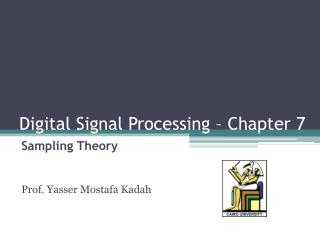DownloadDownload PresentationDigital Signal Processing – Chapter 7

# Digital Signal Processing – Chapter 7

Download Presentation## Digital Signal Processing – Chapter 7

- - - - - - - - - - - - - - - - - - - - - - - - - - - E N D - - - - - - - - - - - - - - - - - - - - - - - - - - -
##### Presentation Transcript

1. Digital Signal Processing – Chapter 7 Sampling Theory Prof. Yasser MostafaKadah

2. Textbook Luis Chapparo, Signals and Systems Using Matlab, Academic Press, 2011.

3. Sampling Motivation • Many of the signals found in biomedical applications are analog • If we wish to process these signals with a computer it is necessary to sample, quantize, and code them to obtain digital signals. • Sampling in time + quantization in amplitude • Sampling issues • How to sample in time (sampling period) • How to quantize in amplitude (number of bits)

4. Uniform Sampling • Sampling function • Periodic sequence of impulses of period Ts • Sampled signal:

5. Uniform Sampling • Fourier Transform of a shifted delta function: • Hence, Period = Ts Period = 2/Ts

6. Uniform Sampling • Sampling in the frequency domain: = Sampling

7. Sampling Rate Calculation • Requirement: No Aliasing

8. Sampling Rate Calculation • Nyquist criterion

9. Sampling Rate Calculation: Example

10. Reconstruction of the Original Continuous-Time Signal from Samples • Assuming band-limited signal and sufficient sampling, original signal can be recovered by low-pass filtering

11. Sampling of Infinite Bandwidth Signals: Anti-Aliasing Filter • Anti-aliasing filter: a low-pass filter applied to the input signal to make sure that the signal to be sampled has a limited bandwidth • Applied in all practical analog-to-digital converters

12. Sampling of Modulated Signals • Nyquist sampling rate condition applies to low-pass or baseband signal x(t) with maximum frequency max • Consider a modulated signal xm(t)= x(t)exp(jct)where x(t)is the baseband signal and exp(jct)is the carrier with carrier frequency c >> max • To avoid aliasing the shifting in frequency should be such that there is no overlapping of the shifted spectra: • Independent of carrier frequency • General case: s> B (Bandwidth of signal)

13. Sampling of Modulated Signals: Illustration s> B Xs() After Sampling B X() 0 0 B

14. Sampling of Modulated Signals: Biomedical Examples • Sampling in Magnetic Resonance Imaging (MRI) • In 1.5T MRI, the signal frequency has a small bandwidth but carried on around 64 MHz • Practical sampling rates used in many commercial systems is only 1 MHz. • Sampling in pulsed-wave Doppler ultrasound (PWD) • The ultrasound center frequency used is around 5-10 MHz and the Doppler shift around that center frequency is only a few kHz. • Practical sampling in PWD is limited by physics to around 5-10 kSa/s and it is usually sufficient

15. Practical Aspects: Quantization and Coding • Amplitude discretization of the sampled signal xs(t)is accomplished by a quantizerconsisting of a number of fixed amplitude levels • Quantization error • Example: Doppler signal sampling • Need at least 12-14 bits per sample • Useful signal superimposed on large clutter can be lost if lower quantization resolution is used

16. Problem Assignments • Problems: 7.1, 7.2, 7.5, 7.6, 7.7, 7.10, 7.11, • Turn in problems marked in red as homework • Partial Solutions available from the student section of the textbook web site